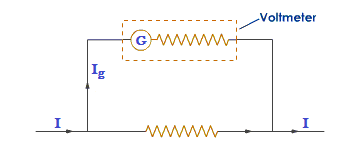# Conversion of Galvanometer into a Voltmeter

A galvanometer is an electrical device which detect the electric current in a circuit.It also gives the direction of flow of current in a circuit. To convert a galvanometer into a voltmeter, a high resistance ‘R’ is connected in a series with the coil of galvanometer.Let ‘G’ be the resistance of the coil of the galvanometer and Ig be the amount of current at which galvanometer gives full scale deflection. Let ‘R’ be the resistance connected in series with a coil of galvanometer, so that the galvanometer becomes a voltmeter of range (0-V) volt.

Now,

$$\text{V = I}_g\text{(R+G)}$$
$$\text{or,}\frac{\text{V}}{\text{I}_g} = \text{R + G}$$
$$\text{or, R} = \frac{\text{V}}{\text{I}_g} – {\text{G}}$$

Let Rnet be the net resistance of the voltmeter.

Then, the effective resistance of the voltmeter is given by:

∴ Rnet = R + G

Since, R is high, the resistance of the voltmeter Rv  is high and it will not draw much current from the circuit.

Do you like this article ? If yes then like otherwise dislike : 6